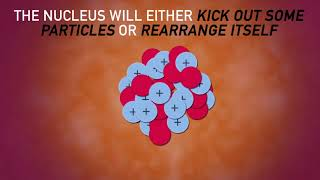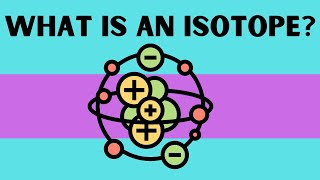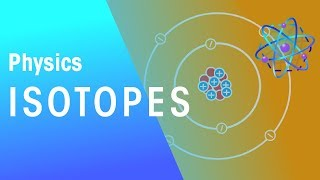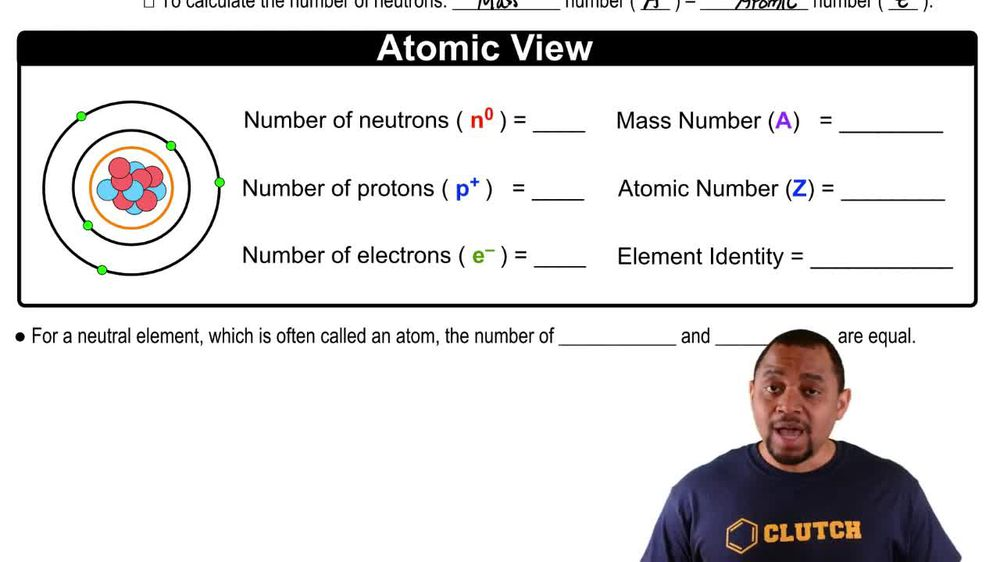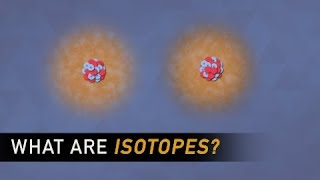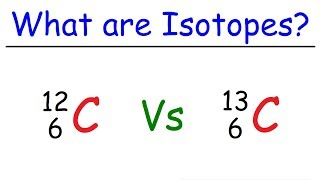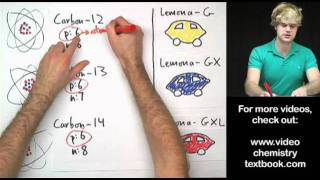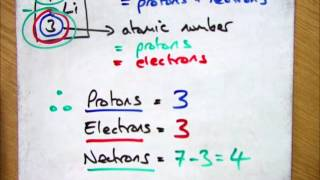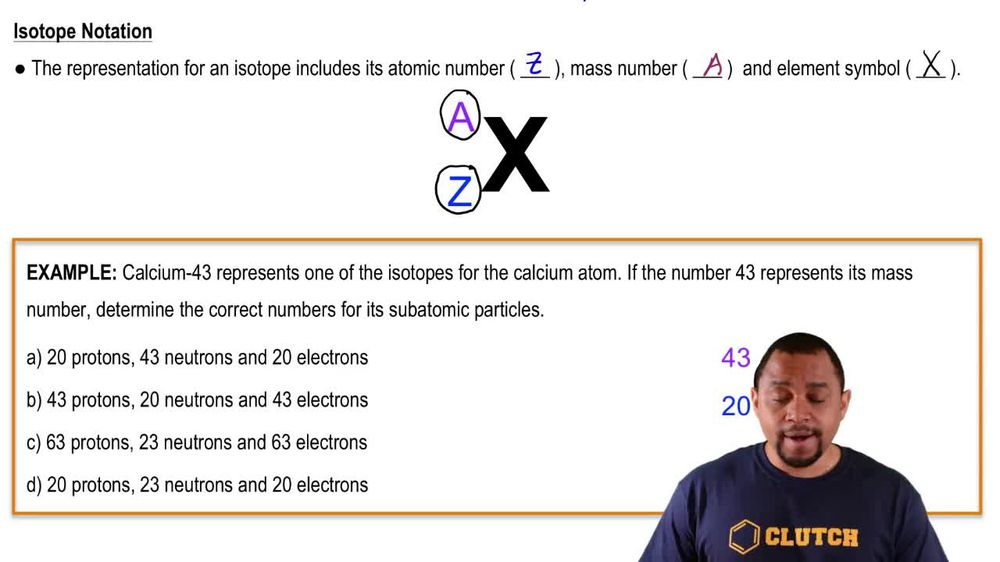Start typing, then use the up and down arrows to select an option from the list.
1. 2. Atoms & Elements2. Isotopes
Problem

# Write symbols for the following isotopes: (a) Z = 58 and A = 140 (b) Z = 27 and A = 60

Relevant Solution2m
Play a video:
Hello everyone today. We have the following problem provide the symbol of the isotopes given below. So for both of these are symbol is going to be represented by X. As our symbol followed by A. And the top left, and Z in the bottom left A. Is going to represent our mass number and Z is going to represent our atomic number. And so what do these mean? So our atomic number is going to be the number of protons of our element. And so our mass number, it's going to be the number of protons plus the number of neutrons in our element. And so now we can begin constructing for both of these symbols. So our atomic number is equal to the number of protons for A. We see that Zs 72. So if you only go on the periodic table, we go to the atomic number 72 we're gonna find half the um or H. F. We're also going to place that 72 at the bottom left and then the mass number at the top left. And so this is going to be our answer for the first part. For the second part we see that we have Z equals 42 or our atomic number equaling 42. And if we go to the periodic table, that's gonna equal molybdenum which is going to be represented by M. O. As our symbol, 42 is our atomic number. That goes in the bottom left and 98 as our mass number goes on the top left. And so with that we have constructed our two symbols for these problems, and with that, I hope this helped, and until next time.